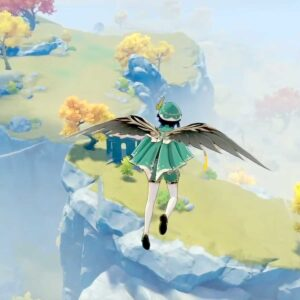# Sphere Of Stars

10 September 2020
3
• ##### TYPE
0
(0)

y p G p h p G p | t p f p G p f p
r I d I f I d I | w y a y S y a y
k j j G k j j G | k j L j k j L j
z k k G z L k G | z L L k j G d f
G h j G k j j G | k j L j z j x j
v C C z z L k g | k z L k j G d f
G | f | d | p | d | S | a | S a p
S f
k j j G k j j G | k j L j k j L j
z k k G z L k G | z L L k j G d f
G h j G k j j G | k j L j z j x j
v C C z z L k g | k z L k j G d f

Rate This Music Sheet:

Average rating 0 / 5. Vote count: 0

No votes so far! Be the first to rate this music sheet.

Thank you for rating this song!

If you have any specific feedback about how to improve this music sheet, please submit this in the box below.

Sphere Of Stars is a song by Hesperidia. Use your computer keyboard to play Sphere Of Stars music sheet on Virtual Piano. This is an Easy song and requires practice. The song Sphere Of Stars is classified in the genre of Classical on Virtual Piano. You can also find other similar songs using Relaxing.

## Related

•8 w u| |8 e I| |8 w u| |8 e y|h|[l8] w u|z x [C8] e I vCx z [x8] w u|z l [z8] e [yj]| |[l8] w u|l z [k8] Qlk[yj]||h [j8] q [xu]| |8 q y|h|[s8]fhlw [ut]|z x [j8]lCe [tI] vCx z [f8]hlxw [ut] z z l [d8]Gjze [yjQ]| |[sq]fjlt [pi]|l xz [wkhf] y [oja]||h [o9]dfje [yI]|||x C [h8]lzvw [ut]|v b [j8]lCe [xtI]|z|8 w [xutkf]|[znkC]|[lC8] evC[xtI]|z|[qg]jlxt [pi]|z l [wkhd] y [oa] lkj h [jfd9] e [yI]| |9 e y|x C [zvlh8] w [ut]|v b [ljC8] e [xtI]|z|8 w [xutokf]|[znC]|[tsljIC] vCx|z|[qg]jlxt [pi] z z l [wkhfd] y [oa] lkj h [pjfed] u d| |S| |[oh]|[tsol]| |[zd] [xf] [tpGC]| |[xf] [zd] [tG][ol]ax| |[zd] [sl] [ztpg]|j| |[tod]hl| |[sl] [zd] [tkdIG]| |[pj] [oh] [tjid]|[xg]|||o|[y8][wu]tos||d f [I8]eptsdG||hGf d [u8][wo]ysf||d s [y81]Ipd [ype]| |[spi84]| |s fd [oa95] sap||o [upe6] u d| |[upeT6]

Level: 7
Length: 01:57
Intermediate

#### Yu-Peng Chen

•[qa] t p t i t [qa] t p t i t [qa] t p t u [yt] [u0] r|r|r [qa] t p t i t [qa] t p t i t [qa] t p t u [yt] [u0] r|r|r [i9] e t e r e [i9] e t e r e [i8] w u w r [we] [r8] w|w|w [i9] e t e r e [i9] e t e r e [i8] w u w [ua] w [uo8] w|w|w [pi^] q [piE] q [ypi] q [pd^] q [pdE] q [ypd]| [uo6] 0 [uoe] 0 [uto] 0 [us6] 0 [wuo] 0 t| [yi6] 9 [yqi] 9 [yiE] 9 [uP6] 9 [uqP] 9 [uPE]| [ut4] 8 [yt0] 8 [tpe] 0 [ut4] 8 0 8 e| [pi^] q [piE] q [ypi] q [pd^] q [pdE] q [ypd]| [uo6] 0 [uoe] 0 [uto] 0 [us6] 0 [wuo] 0 t| [yi7] q [yoe] q [yp] q [ua7] q [usr] q [yud] q [pi7] q [iea] q [yid] e [uf0]|r|u
Level: 5
Length: 00:50
Intermediate

#### Koji Kondo

•[o8]|iu y [t8] y u t [yw]uiy[u8]|y[wt] r [t8]| [o8]|iu y [t8] y u t [yw]uiy[u8]|y[wt] r [t8]| [yw]|ui y [u8]|io y [u8]Io [p9]as [wa] [p9] [wo]| [o8]|iu y [t8] y u t [p8]ppp[o8]|i[wu] y [t8]
Level: 4
Length: 00:32
Easy